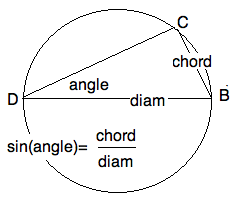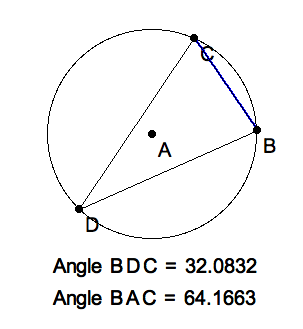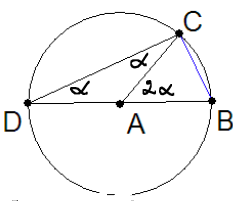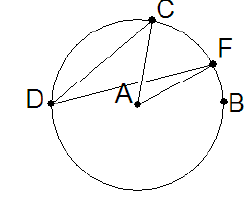## 1. Introduction

In this lesson we

• Remind you of the Law of Sines

• Show how the cross ratio is a perspective invariant

• Apply this to create a ruler for perspective drawings

## 2. The Law of Sines

From high school trigonometry you remember that the sine of an angle of a right triangle is defined as the ratio of the opposite side over the hypotenuse. From the calculus, you will recall that the sine is a differentiable function $\sin(\theta)$ from the reals to the interval $\[-1,1$] equal to the x-coordinate of a point on the unit circle that has travelled a total arclength of $\theta$ units from $(1,0)$. Recall that a line segment cutting across a circle is called a secant, and sometimes it is also called a chord of the circle.The sine of an angle.

The Greeks called the ratio of a secant $BC$ to the diameter $DB$ of a circle the chord of the peripheral angle $\angle BDC$ subtending the secant. As we shall see, this is also the sine of the angle. The word "chord" was translated into Arabic "jiba" ( from Sanscrit "jya" or "bow string") by the scholars in Baghdad who preserved Greek geometry during the Dark Ages. When Gherardo of Cremona (ca 1150) translated geometry from Arabic to Latin, he mistook the word for "jaib" or "bosom, fold in a garment, curve" in Latin, "sinus".Standard Labels

This definition yields an easy proof of the

Proof.

From $\sin \alpha = \frac{a}{d}$ where $d$ is the diameter of the circumcircle $\bigcirc ABC$, we see that the three ratios each equal the reciprocal of the diameter. Hence they are equal to each other.

## 3. Peripheral Angle Theorem

Of course, this proof begs the question: if the angle is transferred to a different circle, then the ratio of its new subtended secant to the new diameter is the same as the old. In particular, we would like to know that given a secant of a circle, every peripheral angle subtending this this secant has the same sine in the traditional sense.Click image to download PAT.seg to explore it in KSEG.
Comment.

We will prove this theorem in 3 steps. The first depends on an older theorem on exterior angles of Euclidean triangles. By "older", we mean it was proved closer to the postulates in Euclid’s Elements. By keeping track of the age of theorems we avoid circular reasoning.

### 3.1. Proof of EXT

You should use ruler and compass or KSEG to draw the figure being described here. We purposely do not include a picture of it so that you can practice following a description of a figure.

Double the median $A A'$ to $E$ beyond the midpoint $A'$ of $BC$, and connect $E$ to $B$. For reference, label a point on $(AB)$ beyond $B$, i.e. so that $B$ is between $A$ and $D$. Because

 $A A' \cong EA'$ $\angle A A'C \cong \angle EA'B$ $C A' \cong BA'$

We have that $\triangle A A' C \cong \triangle EA'B$. Therefore $\angle ACA' \cong \angle EBA'$ and $CA || BE$. But then $\angle BAC \cong \angle DBE$ and we’re done.

Question 1.

What happens if the triangle is isosceles, with $|B-A|=|B-C|$?

The the exterior angle is twice (either) of the opposite interior angles.

### 3.2. Proof of PAT when angle grazes the center

We can imagine the problem to be that of finding a good place to sit in a loge of in a circular theater. La Scala and Schakespeare’s Globe Theater had its loges arranged in a circular wall. By the PAT, every loge occupant saw the stage (a secant) with the same angle, hence the same apparent width. Of course, the distortion could not be avoided, and the queen still sat directly in the royal loge opposite the stage.Let $A$ be the center of the circle, a lie on angle-leg $DB$. By the EXT, the peripheral angle is half the central $\angle BAC$. Since $\triangle DCB$ is inscribed in a semicircle, it is a right triangle, and $\sin D = \frac{CB}{diameter}$, justifying the two Greek definitions of the sine of an angle. It also proves

Corollary.

The length of a secant of a circle is the diameter times the sine of half the central angle it subtends.

### 3.3. Proof of PAT when angle sees the centerClick image to download PAT.seg to explore it in KSEG.

This means, that $A$ lies inside the angle. Draw the diameter $DAE$ and observe that we have two cases of the first case, one above this diameter, and one below. Thus

 $\angle BDC = \angle BDE + \angle EDC = \frac{1}{2} (\angle BAE + \angle EAC) = \frac{1}{2} \angle BAE$Click image to download PATadder.seg which opens in KSEG.
Exercise 1.

Apply a similar argument, with subtraction replacing addition of periferal and central angles for the last case, that the periferal angle does not see the center.So, we have exhaustively proved PAT. You should note that we have used exclusively Euclidean arguments, very possibly the proof that Euclid wrote in his Elements.

Question 2.

What happens when the periferal angle dips behind the stage?

Suddenly the central angle becomes greater than a straight angle. That is, the periferal angle become obtuse. But since the two central angles add up to $2 \pi$, any two periferal angles, one "in front" and one "behind" the secant "stage", become supplementary. But recall, supplementary angles have the same sine.

## 4. The Cross Ratio is a Perspective Invariant

Definition.

The cross ratio, $CR(A,B,C,D$, of four (distinct) collinear points, is $CR(A,B,C,D) = \frac{A-B}{A-D} \frac{C-D}{C-B}$

Definition.

The cross ratio, $CR(a,b,c,d)$, of four (distinct) concurrent lines, is $CR(a,b,c,d) = \frac{\sin \angle ab}{\sin \angle ad} \frac{\sin \angle cd}{\sin \angle cb}$

Note the direction in which we measure a segment matters here, $\frac{A-B}{A-D} = - \frac{B-A}{A-D}$, and so does the orientation in which we measure an angle, $\sin \angle ab = - \sin \angle ba$. However, for two crossing lines, it doesn’t matter which of the two angles we use; being supplementary, their sins are the same. For notational symmetry, we have measured the angles clock-wise. It doesn’t matter, as long as are consistent. Changing the sign of an angle changes the sign of its sin, but not the ratio of two angles whose sign was reversed.

See how it would have been better if Gherardo had used the word "chord". On the other hand, "cochord" might sound worse than "cosine".### 4.1. Proof of CRT

First, we abbreviate $sin AB = \sin \angle APB = \sin \angle ab$ etc. That would be the angles at $P$. Second, we abbreviate $\sin B = \sin \angle PBA = \sin \angle CBP$ because these two angles are supplementary, and so have the same sines. Finally, we show that two numbers are equal by showing that their ratios equals $1$.

 $\frac{CR(A,B,C,D)}{CR(a,b,c,d)}$ $= \frac{ \frac{A-B}{A-D} \frac{C-D}{C-B} }{ \frac{sin AB}{sin AD}\frac{sin CD}{sin CB} }$ $= \frac{ \frac{A-B}{sin AB} \frac{C-D}{sin CD} }{ \frac{A-D}{sin AD}\frac{C-B}{ sin CB} }$ $= \frac{ \frac{P-A}{sin B} \frac{P-C}{sin D} }{ \frac{P-A}{sin D}\frac{P-C}{ sin B} }$ $= \frac{ \frac{P-A}{P-A} \frac{P-C}{P-C} }{ \frac{sin B}{sin D}\frac{sin D}{sin B} }$ $= 1$.

The rearrangement of the fractions has to be undertood carefully. Since everything is happening on the same line, we have replaced the vector $A-D$ by its signed length (the direction matters!). Now we apply the Law of Sines judiciously. Can you find the four triangles in which we replace ratios? This time we are allowed to replace vectors by their signed length because all four are concurrent. This is a little subtle, but has to be said here for completeness.

Comment.

Pretty neat, huh? Mathematicians die for clever arguments like this. The alternative is a considerable longer. While each step of the un-clever argument might be easier to see, there are so many of them that you loose the forest for the trees.

Two sets of four collinear points, $(ABCD), (A'B'C'D')$ are said to be in perspective position, if the four lines $(A'A) (B'B) (C'C) (D'D)$ are concurrent. s

### 4.2. Proof of the PIT

With $a=(A'A)$ etc, observe that

 $CR(A,B,C,D) = CR(a, b, c, d) = CR (A',B',C',D')$.

## 5. Perspective Rulers

By a ruler we mean a line with a copy of the real numbers marked on it. Recall from high school, that $\ell = (AB)$ can be given a ruler by assignin $A |-> 0$ and $B |-> 1$. In particular, we can find all multiples and fractions of unity by ruler and compass. Multiples are easy.

Question 3.

How would you subdivide the segment $AB$ in 7 equal pieces?

If instead of 7 we wanted some power of 2, then taking repeated halves would solve our problem. In fact, by just marking fractions with powers of two in their denominator (think of the inch-system) would work quite nicely in the real world. Such fractions are also called binary fractions. We can approximate every real number by a sequence of convergent binary fractions.

But to get an accurate, and for that matter quicker, answer we can use a similarity theorem from high school geometry. You’ll need pencil and paper to follow along.

On another line $AC$ extend $AC$ seven times to $D$. Now $AC = \frac{1}{7} AD$. Now draw $BD$ and a line parallel to $BD$ through every multiple of $AC$ on the helper line. These mark off seven equal pieces on the given segment $AB$.

Comment.

The previous construction is actually a case of PIT, if we understand mutually parallel as concurrent at infinity. Although it is true, we have not proved PIT for this extended case.

Now, finally, consider a ray in the canvas at $V$ and two points, $Q I$ on the ray. Thus in the Euclidean plane of the canvas $(QIV)$. This represents an infinite line in space, but in perspective. Equally spaced points on the space line would not be drawn with equally spaced points in perspective. The points have to get more and more close to each other as they approach the vanishing point $V$.

To find these milestones you may choose any convenient point $P$ in the canvas, but not on the line $(QI)$. We shall call $P$ a lens. Now choose any other line parallel $s$ to $(PV)$ that is convenient to work with. We shall call $s$ a scale for the perspective line $(VIQ)$.

Now transfer $Q, I$ to $s$ by drawing lines through $P$. That is $Q' = (QP)s \and I' = (IP)s$.

Question 4.

Calculate $CR(X,Q,I,V) = CR(X',Q',I',V')$ ?

$\frac{X-Q}{X-V} \frac{I-V}{I-Q} = \frac{X'-Q'}{X'-V'} \frac{I'-V'}{I'-Q'}$
Notice the $V'$ does not exist. The line $(PV)$ is parallel to the scalin line $s$ and so meets it at $\infty$. If we identify $X' = x, Q' = 0, I' = 1$ then we should also let $V'= \infty$. Then the cross ratio becomse $\frac{x-0}{x-\infty}\frac{1-\infty}{1-0} = x$. This applies a perspective ruler on the perspective line $(OQV)$. Therefore, for two points $X(QV) \and Y(QV)$ we can assign the perspective distance $dist_{QV}(XY) = CR(X,Q,Y,V) = \frac{X'-Q'}{Y'-Q'}$.